## 3.13Limiting multiple components

The calculation and application of a limiter is introduced in Sec. 3.11 for advection of a single scalar property. The ﬁgure below examines when the advected property is a vector, e.g.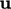in the momentum conservation Eq. (2.47 ).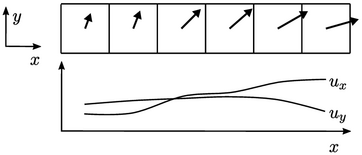It shows a 2D cut along the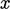-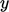plane through a selection of cells in a regular mesh with the velocity vector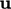displayed for each cell. Whenis interpolated to faces along the-direction using a limited scheme, the simplest approach is to calculate a limiter for each vector component, e.g.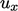, and interpolate the component with that limiter.

In the example above, the proﬁles ofand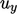in the-direction are quite diﬀerent, so the calculated limiters will be diﬀerent for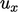and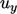components at each face. The limiting will not be invariant under a rotation of the co-ordinate axes, leading to a diﬀerent solution depending on the initial orientation of the geometry with respect to the axes.

The limiting can be invariant using a single limiter calculated from the magnitude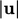, which is applied to all components of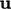. The strength of the limiting corresponds to an average across the components, which is usually insuﬃcient for the component which requires strongest limiting. This can cause instability.

Instead, the ‘V’ scheme calculates the limiter based on the ‘worst-case’ direction, i.e. the direction of steepest gradient in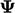at the cell face. It uses the following expression for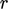for a vector, replacing Eq. (3.12 ) for a scalar: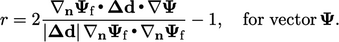(3.17)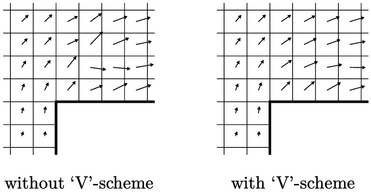While the V scheme ensures invariance, it also provides greater stability than component limiting. It can remove oscillations in solutions, e.g. in the example above of supersonic ﬂow over a step showing the eﬀect in velocity in the cells adjacent to the step corner.

### Multivariate limiting

Multivariate limiting applies the same limiter for advection discretisation across a set of 2 or more equations. It works by calculating the limiter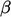for each solution variable in the equation set and applying the lowestto all equations.

It can be used in order to maintain consistency in the transport of individual ﬂuid species, such as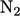,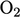, e.g. in the propagation of laminar ﬂame (which is beyond the scope of this book).

Notes on CFD: General Principles - 3.13 Limiting multiple components